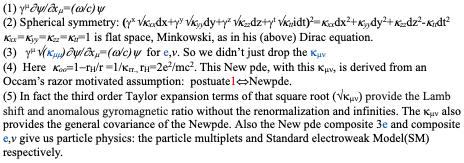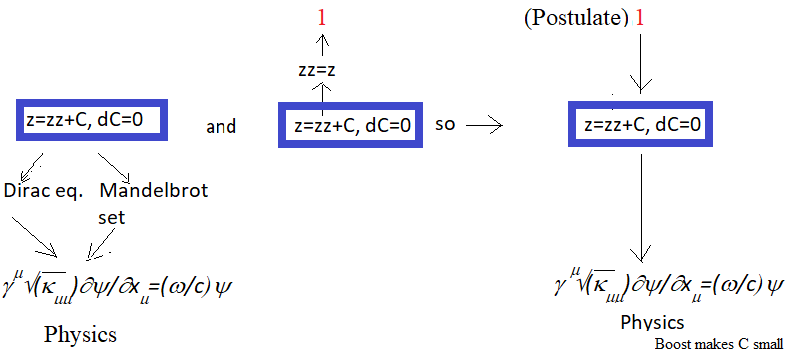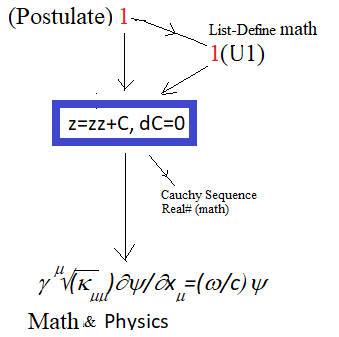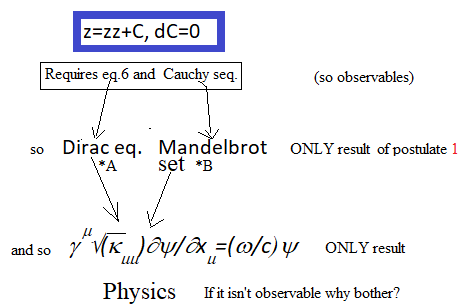# Generally Covariant Generalization of the Dirac equation that does not require gauges

Postulate 1 . z=zz (algebraic definition of 1)

Dirac in 1928 made his equation(1) flat space(2). But space is not in general flat, there are forces.

So over the past 100 years people have had to try to make up for that mistake by adding adhoc convoluted gauge force after gauge force until theoretical physics became a mass of confusion, a train wreck, a junk pile. So all they can do for ever and ever is to rearrange that junk pile with zero actual progress in fundamental theoretical physics being made,.. forever.

By the way note that Newpde (3)

is NOT flat space (4) so it cures this problem(5).

References(4)  Here  koo=1-rH/r =1/krr,  rH =(2e2)(1040N) /(mc2). The N=..-1,0,1,.. applies to the Nth Fiegenbaum point self-similar fractal scale. Newpde from Occam’s razor optimized postulate1:                                                                                   z=zz is the algebraic definition of 1 and adding (small) constant C is trivial in z=zz+C, dC=0 (1)A  Substitute  z=1+dz into equation 1 and get  2D Dirac equations for e,v (sect.1.1).B  Substitute left side z into right side zz repeatedly in equation 1and get the 2D Mandelbrot setA,B together gives 4D Newpde.  So Postulate1->Newpde

Introduction

Part I     1U1 states

Part II    1U1U1 states

Part III   Mixed State

Rest       Miscellaneous

Figures:

Figure 1 solves the puzzle of physics and real#math (just postulate 1) and figure 5 explains why.

(Ockam’s razor motivated) Postulate 1 <->Physics   :Equation 1 z=zz+C, dC=0 gets the New pde and physics (left side of fig.1)and yet the z=zz algebraic definition of 1 is also the small C limit of equation 1 (middle of fig.1). So eq.1 hints strongly that the (Ockam’s razor motivated) Postulate 1->Physics   is correct (right side of fig.1). fig.1See sect.4 of Postulate 1 file above implies real#math. fig.2Uniqueness: Need at least some measurable “observables” (Hermitian operator fig.3 formalism eq.6) and Cauchy sequence of rational numbers to derive real#(eigenvalues) math as well. So New pde is the ONLY result of postulate 1.

Mandelbrot Set

Plug in the left side (of eq.1) z into the right side zz repeatedly and use  δC=0 and get the Mandelbrot set iteration formula.                                                                                               The Mandelbrot set Cis (and from the postulated  δCM=0)zN+1=zNzN+CM (since  δ(z’-zz)=  δ(zN+1-zNzN)= δ(infinity-infinity)=0). zo=0. Fiegenbaum point CM smallest real line Mandelbulb on next smaller (baseline) scale. Mandelbulb areas (drdt) for smallest Clifford algebra extremum drdt.

fig.4

Boost  m1=tau+mu+me=3e from S=½ to L=1 at stable r=rH, 2P3/2 with m1/2=mreduced mass. See above sect.2 of above postulate 1 file for some more applications. See PartI, partII, PartIII for the rest.

Comparison and contrast with mainstream concerns:

The origin of the many postulates of quantum mechanics is considered to be a mystery by one and all.

Not really:  1) Quantum mechanics arises from a circle.(see below)

The connection between general relativity and quantum mechanics is stated over and over again to be the greatest unsolved problem in physics.

Not really:  2) General Relativity(GR) comes out of quantum mechanics in 1 step.(see below)

A century has been devoted to the origin of high energy particle physics by thousands of people using billions of dollars worth of particle accelerators. It is supposed to be quarks, gluons, qcd SU(3) gauge forces, require many free parameters,..and so on and so forth.

Not really:  3) (high energy 3e composite) Particle physics is just a ortho and para state (see below)

The latest version “Standard electroweak Model” is now encumbered by pages of Lagrangian density terms, and so literally hundreds of postulates.

Not really: 4) Rotate e,v to get the Standard electroweak Model (it’s merely composite e,v). (see below)